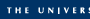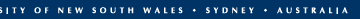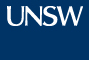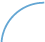Contacts Library myUNSW WebCTEFTSL - Equivalent Full-time Student Load
EFTSL - Equivalent Full-time Student Load

Equivalent full-time student load (EFTSL) is a measure of a student's study load. An EFTSL of 1.0 is the standard annual study load of a student undertaking a program on a full-time basis.

Calculating Student Contribution Amounts and EFTSL

At UNSW, a normal full-time enrolment for one year is defined as 48 units of credit (24 per session). A course (unit of study, e.g. MATH1011) has the same unit of credit value and generates the same load (EFTSL) irrespective of the program (e.g. BSc) or the stage in which it is taken. Most courses at UNSW have a value of 6 unit of credit (6UOC).

To calculate the EFTSL of a course, you will need to note its units of credit (UOC) value. The unit of credit value for a course is displayed in the Online Handbook.

Courses with a UOC value of...
Courses with a UOC value of...
6 UOC divided by 48 (total no. of units for a full-time year) = 0.125 EFTSL
3 UOC divided by 48 (total no. of units for a full-time year) = 0.0625 EFTSL
8 UOC divided by 48 (total no. of units for a full-time year) = 0.167 EFTSL

Example:
Ana commences a Bachelor of Commerce in 2007. In her first year she enrols in 5 Commerce courses (each 6 UOC) and 3 Arts courses (each 6 UOC), and thus undertakes a standard annual full-time workload of 48 units of credit.

Her 2007 student contribution amount will be calculated as follows:

Example
Example
Commerce
\$7,118 x (6 UOC divided by 48 UOC = 0.125 EFTSL) x 5 = \$4,448.00
Commerce cost, Band 2 multiplied by EFTSL value for the course multiplied by no. of courses
Arts
\$4,996 x (6 UOC divided by 48 UOC = 0.125 EFTSL) x 3 = \$1,1873.00
Arts cost, Band 1 multiplied by EFTSL value for the course multiplied by no. of courses
= \$6,322.00
Total payable
Multiply the total amount by 80% to calculate the up-front payment option
Australian citizens and holders of a permanent humanitarian visa receive a 20% discount for full up-front payment or partial payments of \$500 or more.